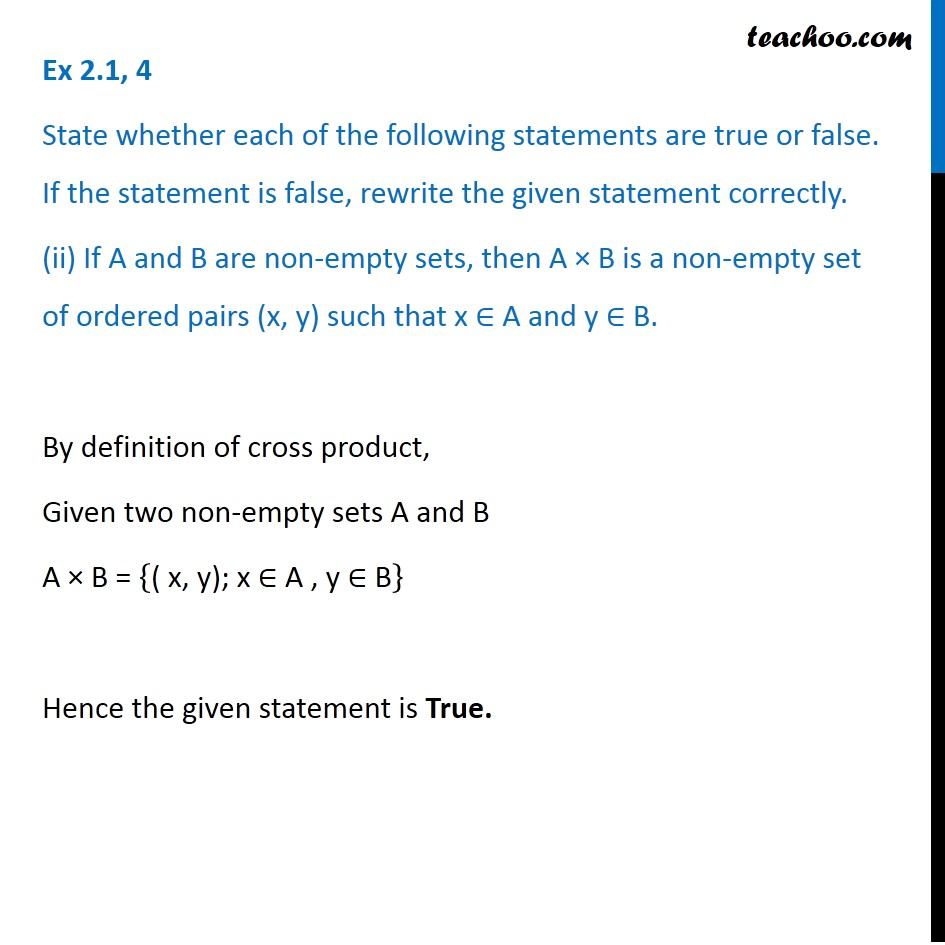Ex 2.1

Chapter 2 Class 11 Relations and Functions
Serial order wiseLearn in your speed, with individual attention - Teachoo Maths 1-on-1 Class

### Transcript

Ex 2.1, 4 State whether each of the following statements are true or false. If the statement is false, rewrite the given statement correctly. (ii) If A and B are non-empty sets, then A × B is a non-empty set of ordered pairs (x, y) such that x ∈ A and y ∈ B. By definition of cross product, Given two non-empty sets A and B A × B = {"( x, y); x ∈ A , y ∈ B" } Hence the given statement is True.